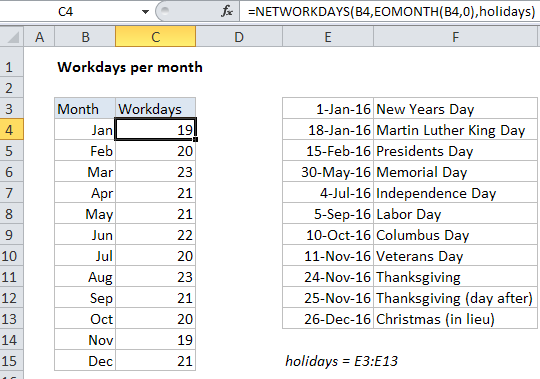## Excel Office

Excel How Tos, Tutorials, Tips & Tricks, Shortcuts

# How to calculate workdays per month in Excel

To calculate workdays per month, use the EOMONTH function together with the NETWORKDAYS function.

## Formula

`=NETWORKDAYS(date,EOMONTH(date,0),holidays)`## Explanation

In the example shown, the formula in C4 is:

`=NETWORKDAYS(B4,EOMONTH(B4,0),holidays)`

Where “holidays” is the named range E3:E13.

### How this formula works

First, it’s important to understand that the values in the Month column (B) are actual dates, formatted with the custom number format “mmm”.

Worked Example:   Sum by month in Excel

For example, B4 contains January 1, 2014, but displays only “Jan” per the custom number format.

The formula itself is based on the NETWORKDAYS function, which returns the number of working days between a start date and end date, taking into account holidays (if provided).

Worked Example:   Count dates in current month in Excel

For each month, the start date comes from column B and the end date is calculated with the EOMONTH function like so:

`EOMONTH(B4,0)`

EOMONTH takes a date and returns the last day of a month. The month itself is controlled by the 2nd argument. Since in this case we want to stay in the same month, we use zero.

Worked Example:   How to get Weekdays, Working days between Two Dates in Excel

Finally, a list of holidays is provided as the 3rd argument to NETWORKDAYS using the named range holidays (E3:E13).

With this information, NETWORKDAYS calculates the number of working days in each month, automatically excluding weekends and holidays.